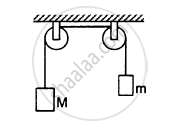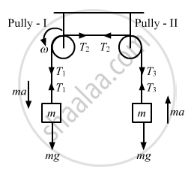Advertisement Remove all ads

# The Pulleys Shown in the Following Figure Are Identical, Each Having a Radius R And Moment of Inertia I. Find the Acceleration of the Block M. - Physics

Sum

The pulleys shown in the following figure are identical, each having a radius R and moment of inertia I. Find the acceleration of the block M.Advertisement Remove all ads

#### Solution

Free the body diagram of the system,For block of mass M,

$Mg - T_1 = Ma ..........(1)$

$\left( T_1 - T_2 \right) R = I\alpha\text{ using, }a =\alpha r$

$\Rightarrow \left( T_1 - T_2 \right) = I\frac{a}{R^2}......(2)\left(\text{For pully 1} \right)$

$\text{Similarly, }\left( T_2 - T_3 \right) = I\frac{a}{R^2}..........(3)\left(\text{For pully 2}\right)$

For block of mass m,

$T_3 - mg = ma.........(4)\left(\text{For block m} \right)$

Adding equations (2) and (3), we get

$\left( T_1 - T_3 \right) = \frac{2Ia}{R^2}..........(5)$

Adding equations (1) and (4), we get

$- mg + Mg + \left( T_3 - T_1 \right) = Ma + ma..........(6)$

Using equations (5) and (6), we get

$Mg - mg = Ma + ma + \frac{2Ia}{R^2}$

$\Rightarrow a = \frac{\left( M - m \right)g}{\left( M + m + \frac{2I}{R^2} \right)}$

Is there an error in this question or solution?
Advertisement Remove all ads

#### APPEARS IN

HC Verma Class 11, 12 Concepts of Physics 1
Chapter 10 Rotational Mechanics
Q 36 | Page 197
Advertisement Remove all ads

#### Video TutorialsVIEW ALL 

Advertisement Remove all ads
Share
Notifications

View all notifications

Forgot password?